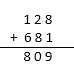# ICSE Class 8 Maths Selina Solutions Chapter 5 Playing with Number

The Class 8 Maths Chapter “Playing with Number” revolves around numbers which makes the chapter more interesting. Mathematics is a world of numbers where one can simply play with the numbers and can easily discover various analogies and relations of the numbers from the number line. A number is said to be in a generalized form if it is expressed as the sum of the product of its digits with their respective place values. For eg: 56 = 10 x 5 + 6, 37 = 10 x 3 + 7, 90 = 10 x 9 = 0, etc. The chapter also explains about deducing the divisibility test rules for a two or three-digit number expressed in the general form.

The Selina Solutions of Class 8 Maths Chapter 5 Playing with Numbers are provided here to help the students to clear all their doubts easily and work on the weak points. These solutions are comprehensive and given in a step by step format.

## Download ICSE Class 8 Maths Selina Solutions PDF for Chapter 5:-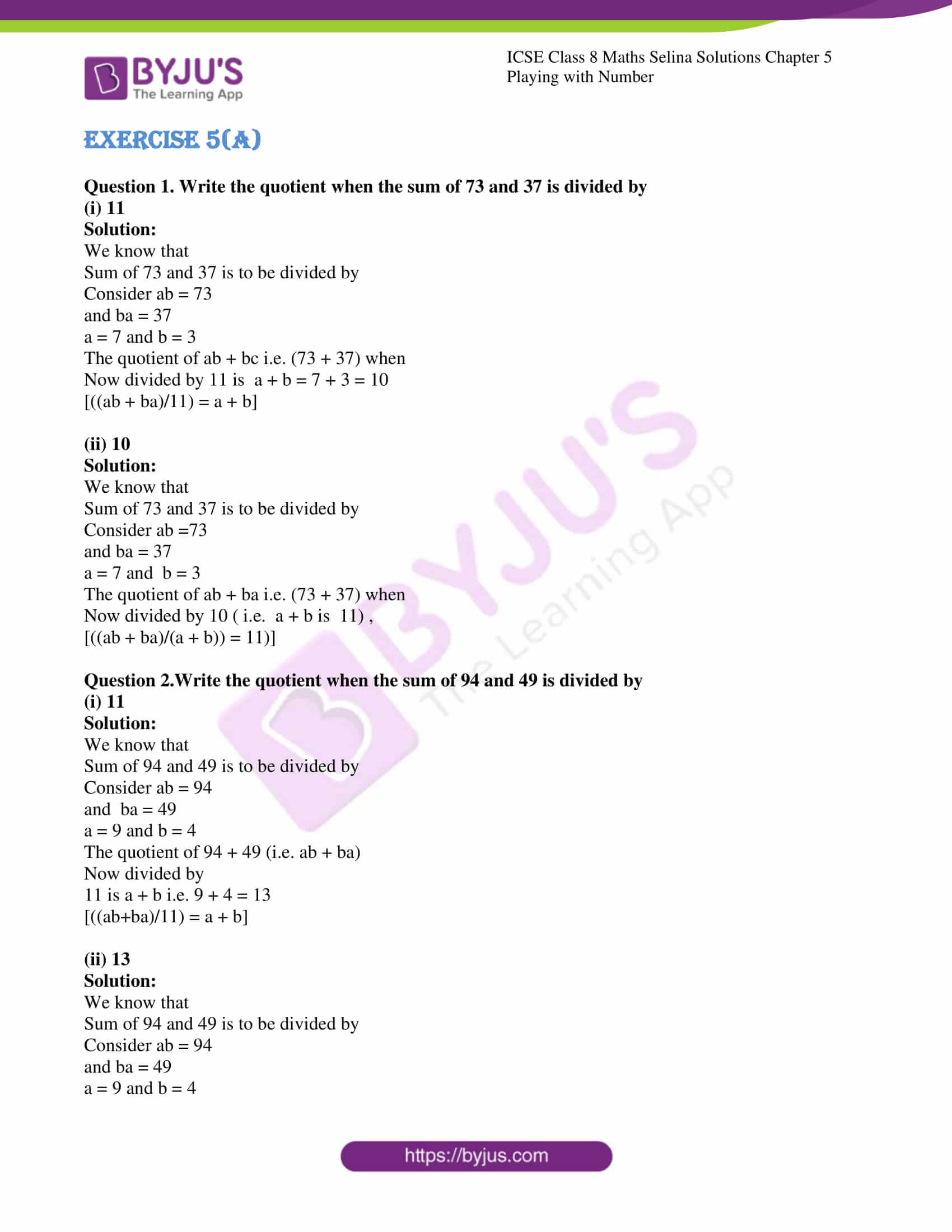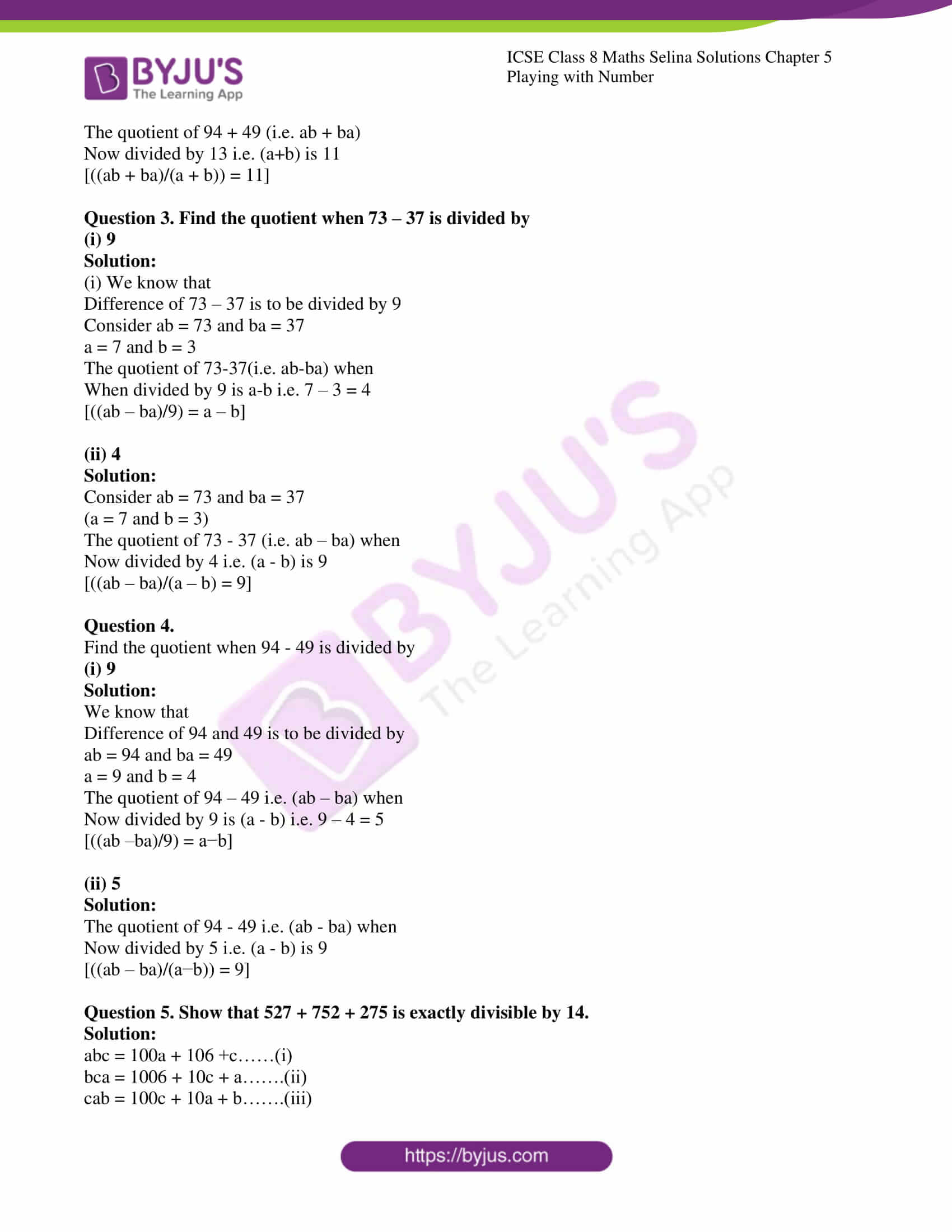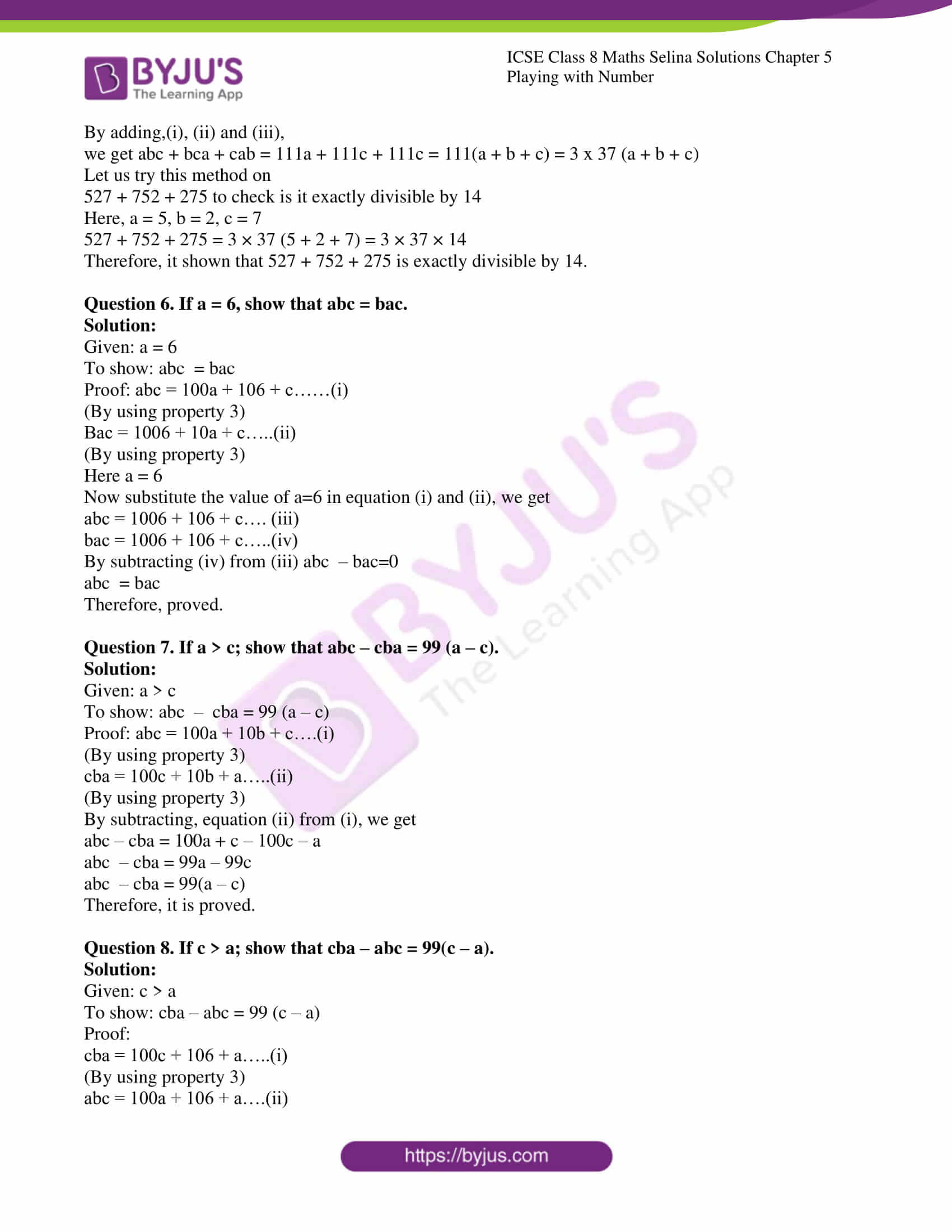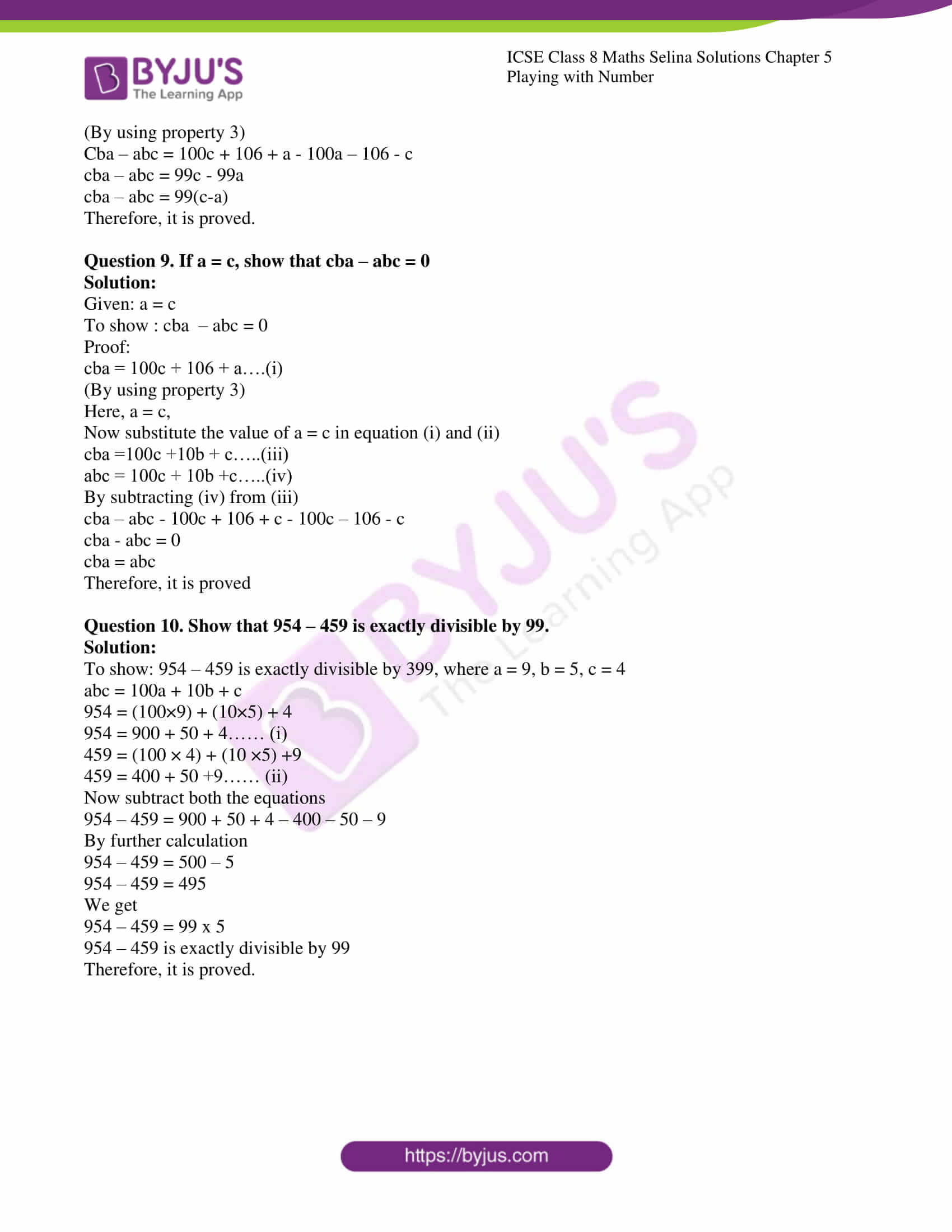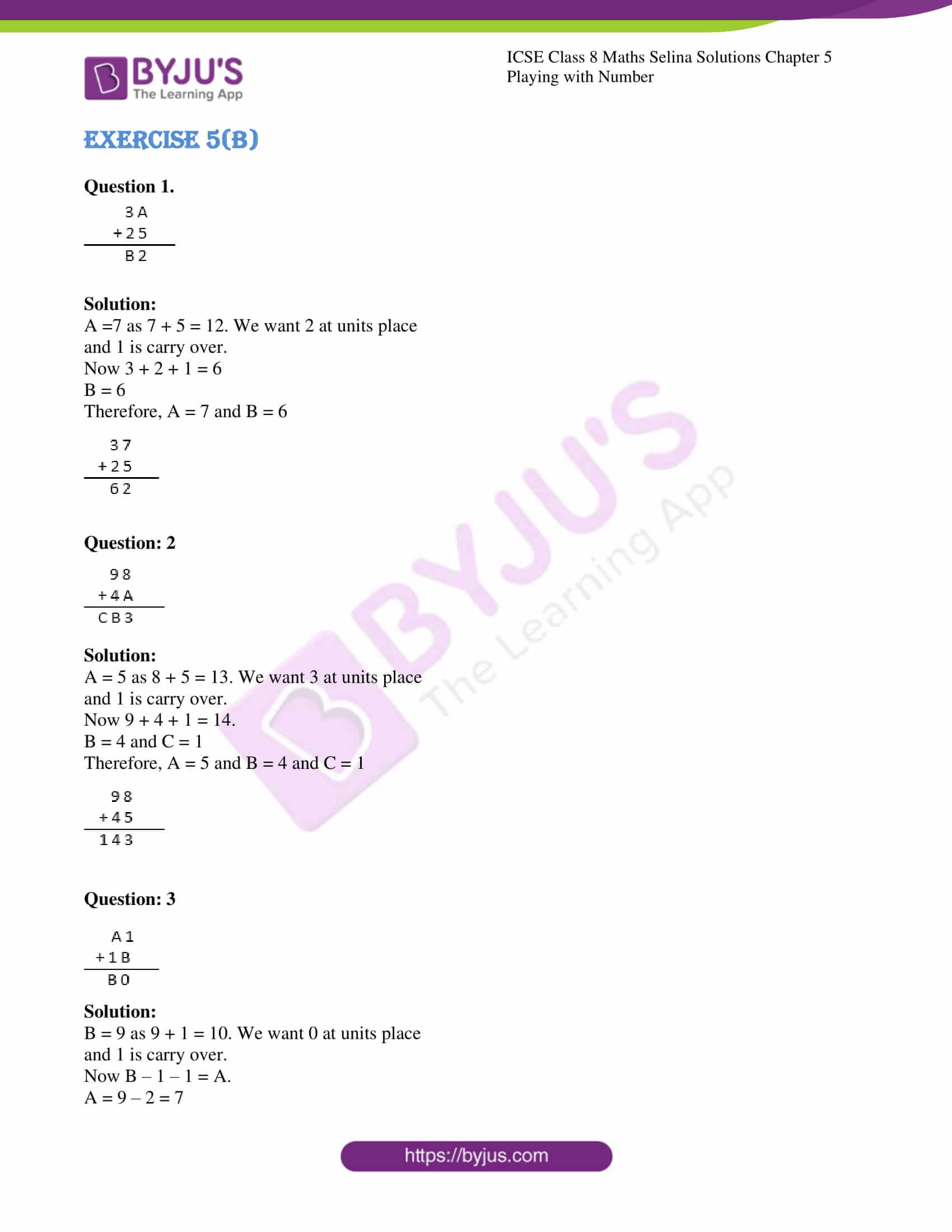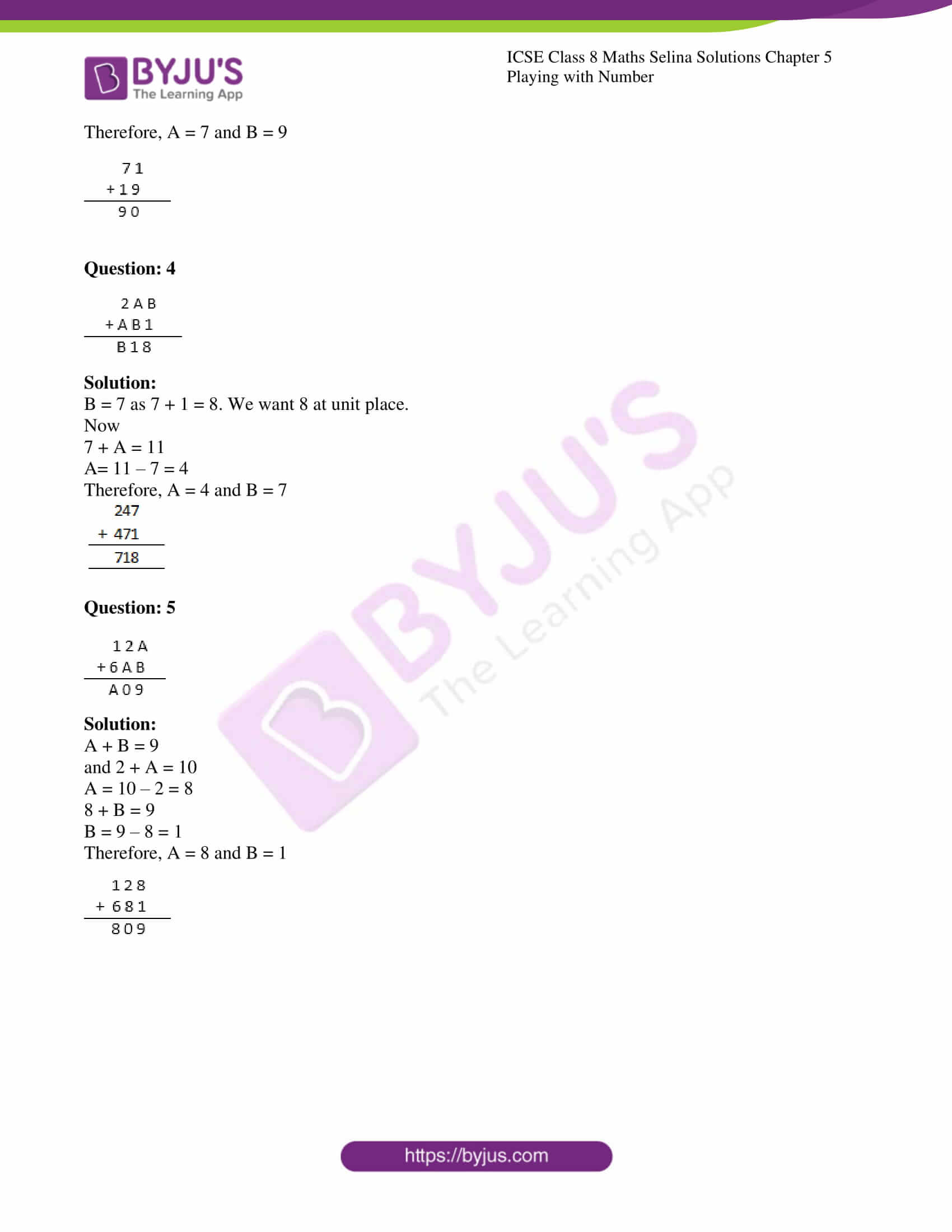### ICSE Class 8 Maths Selina Solutions Chapter 5 Playing with Number – Exercise 5 (A)

Question 1. Write the quotient when the sum of 73 and 37 is divided by

(i) 11

Solution:

We know that

Sum of 73 and 37 is to be divided by

Consider ab = 73

and ba = 37

a=7 and b=3

The quotient of ab+bc i.e. (73+37)when

Now divided by 11 is  a+b=7+3=10

$$\begin{array}{l}\left(\frac{a b + b a}{11}=a+b\right) \end{array}$$

(ii) 10

Solution:

We know that

Sum of 73 and 37 is to be divided by

Consider ab=73

and ba=37

a=7 and  b=3

The quotient of ab+ba i.e. (73 + 37) when

Now divided by 10 ( i.e.  a + b is  11) ,

$$\begin{array}{l}\left(\frac{a b+b a}{a+b}=11\right) \end{array}$$

Question 2.Write the quotient when the sum of 94 and 49 is divided by

(i) 11

Solution:

We know that

Sum of 94 and 49 is to be divided by

Consider ab=94

and  ba=49

a=9 and b=4

The quotient of 94+49 (i.e. ab + ba)

Now divided by

11 is a+b i.e. 9 + 4 = 13

$$\begin{array}{l}\left(\frac{a b+b a}{11}=a+b\right) \end{array}$$

(ii) 13

Solution:

We know that

Sum of 94 and 49 is to be divided by

Consider ab = 94

and ba = 49

a = 9 and b = 4

The quotient of 94+49 (i.e. ab+ba)

Now divided by 13 i.e. (a+b) is 11

$$\begin{array}{l}\left(\frac{a b+b a}{a+b}=11\right) \end{array}$$

Question 3. Find the quotient when 73 – 37 is divided by

(i) 9

Solution:

(i) We know that

Difference of 73 – 37 is to be divided by 9

Consider ab=73 and ba=37

a=7 and b=3

The quotient of 73-37(i.e. ab-ba) when

When divided by 9 is a-b i.e. 7-3=4

$$\begin{array}{l}\left(\frac{a b-b a}{9}=a-b\right) \end{array}$$

(ii) 4

Solution:

Consider ab=73 and ba=37

(a=7 and b=3)

The quotient of 73-37 (i.e. ab – ba) when

Now divided by 4 i.e. (a-b) is 9

$$\begin{array}{l}\left(\frac{a b-b a}{a-b}=9\right) \end{array}$$

Question 4.

Find the quotient when 94-49 is divided by

(i) 9

Solution:

We know that

Difference of 94 and 49 is to be divided by

ab = 94 and ba = 49

a = 9 and b = 4

The quotient of 94 – 49 i.e. (ab – ba) when

Now divided by 9 is (a-b) i.e. 9 – 4 = 5

$$\begin{array}{l}\left(\frac{a b-b a}{9}=a-b\right) \end{array}$$

(ii) 5

Solution:

The quotient of 94-49 i.e. (ab-ba) when

Now divided by 5 i.e. (a-b) is 9

$$\begin{array}{l}\left(\frac{a b-b a}{a-b}=9\right) \end{array}$$

Question 5. Show that 527 + 752 + 275 is exactly divisible by 14.

Solution:

abc = 100a+106+c……(i)

bca = 1006+10c+a…….(ii)

cab = 100c+10a+b…….(iii)

we get abc + bca + cab = 111a + 111c + 111c = 111(a + b + c) = 3 x 37 (a + b + c)

Let us try this method on

527 + 752 + 275 to check is it exactly divisible by 14

Here, a = 5, b = 2,c = 7

$$\begin{array}{l}527+752+275=3 \times 37(5+2+7)=3 \times 37 \times 14\end{array}$$

Therefore, it shown that 527 + 752 + 275 is exactly divisible by 14.

Question 6. If a = 6, show that abc = bac.

Solution:

Given: a = 6

To show: abc  = bac

Proof: abc = 100a + 106 + c……(i)

(By using property 3)

Bac = 1006 + 10a + c…..(ii)

(By using property 3)

Here a = 6

Now substitute the value of a=6 in equation (i) and (ii), we get

abc = 1006 + 106 + c…. (iii)

bac = 1006 + 106 + c…..(iv)

By subtracting (iv) from (iii) abc  – bac=0

abc  = bac

Therefore, proved.

Question 7. If a>c; show that abc – cba = 99 (a – c).

Solution:

Given: a>c

To show: abc  –  cba = 99 (a – c)

Proof: abc = 100a + 10b + c….(i)

(By using property 3)

cba = 100c + 10b + a…..(ii)

(By using property 3)

By subtracting, equation (ii) from (i), we get

abc – cba = 100a + c – 100c – a

abc  – cba = 99a – 99c

abc  – cba = 99(a – c)

Therefore, it is proved.

Question 8. If c>a; show that  cba – abc = 99(c – a).

Solution:

Given: c>a

To show: cba – abc = 99 (c – a)

Proof:

cba = 100c + 106 + a…..(i)

(By using property 3)

abc = 100a + 106 + a….(ii)

(By using property 3)

cba-abc=100c+106+a-100a-106-c

cba-abc=99c-99a

cba-abc=99(c-a)

Therefore, it is proved.

Question 9. If a = c, show that cba – abc = 0

Solution:

Given: a=c

To show : cba  – abc = 0

Proof:

cba = 100c + 106 + a….(i)

(By using property 3)

Here, a = c,

Now substitute the value of a = c in equation (i) and (ii)

cba =100c +10b + c…..(iii)

abc = 100c + 10b +c…..(iv)

By subtracting (iv) from (iii)

cba-abc-100c+106+c-100c-106-c

cba-abc = 0

cba = abc

Therefore, it is proved

Question 10. Show that 954 – 459 is exactly divisible by 99.

Solution:

To show: 954 – 459 is exactly divisible by 399, where a = 9, b = 5, c = 4

abc = 100a + 10b + c

954=100×9+10×5+4

954=900+50+4…… (i)

459=100×4+10×5+9

459=400+50+9…… (ii)

Now subtract both the equations

954 – 459 = 900 + 50 + 4 – 400 – 50 – 9

By further calculation

954 – 459 = 500 – 5

954 – 459 = 495

We get

954 – 459 = 99 x 5

954 – 459 is exactly divisible by 99

Therefore, it is proved.

### ICSE Class 8 Maths Selina Solutions Chapter 5 Playing with Number – Exercise 5 (B)

Question 1.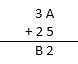Solution:

A=7 as 7+5=12. We want 2 at units place

and 1 is carry over.

Now 3+2+1=6

B=6

Therefore, A=7 and B=6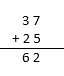Question: 2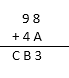Solution:

A=5 as 8+5=13. We want 3 at units place

and 1 is carry over.

Now 9+4+1=14.

B=4 and C=1

Therefore, A=5 and B=4 and C=1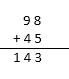Question: 3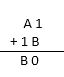Solution:

B=9 as 9+1=10. We want 0 at units place

and 1 is carry over.

Now B-1-1=A.

A=9-2=7

Therefore, A=7 and B=9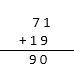Question: 4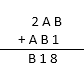Solution:

B=7 as 7+1=8. We want 8 at unit place.

Now

7+A=11

A=11-7=4

Therefore, A=4 and B=7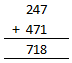Question: 5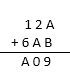Solution:

A+B=9

and 2+A=10

A=10-2=8

8+B=9

B=9-8=1

Therefore, A=8 and B=1